# Class 10 RD Sharma Solutions – Chapter 5 Trigonometric Ratios – Exercise 5.1 | Set 1

• Last Updated : 21 Feb, 2021

### (i) sinA = 2/3

Solution:

sinA = 2/3 = Perpendicular/Hypotenuse

Attention reader! All those who say programming isn't for kids, just haven't met the right mentors yet. Join the  Demo Class for First Step to Coding Coursespecifically designed for students of class 8 to 12.

The students will get to learn more about the world of programming in these free classes which will definitely help them in making a wise career choice in the future.

Draw a right-angled △ABC in which ∠B is = 90°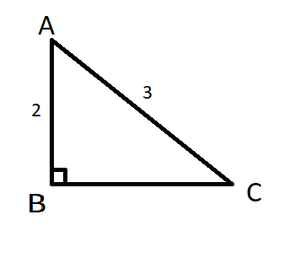Using Pythagoras Theorem, in △ABC,

(Hypotenuse)2 = (Perpendicular)2 + (Base)2

AC2 = AB2 + BC2

(3)2 = (2)2 + (BC)2

9 = 4 + BC

BC2 = 9 – 4 = 5

BC = √5 units

Now,

cosA = Base/Hypotenuse = BC/AC = √5/3

tanA = Perpendicular/Base = AB/BC = 2/√5

cotA = 1/tanA = √5/2

secA = 1/cosA = 3/√5

cosecA = 1/sinA = 3/2

### (ii) cosA = 4/5

Solution:

cosA = 4/5 = Base/Hyptenuse

Draw a right-angled △ABC in which ∠B is = 90°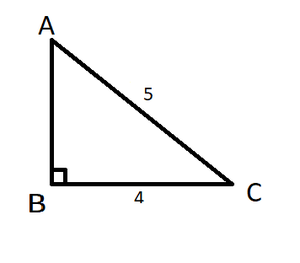Using Pythagoras Theorem, in △ABC,

(Hypotenuse)2 = (Perpendicular)2 + (Base)2

AC2 = AB2 + BC2

(5)2 = (AB)2 + (4)2

25 = AB2 + 16

AB2 = 25 – 16 = 9

AB = √9

= 3 units

Now,

sinA = Perpendicular/Hypotenuse = AB/AC =3/5

tanA = Perpendicular/Base = AB/BC = 3/4

cotA = 1/tanA = 4/3

secA = 1/cosA = 5/4

cosecA = 1/sinA =5/3

### (iii) tanθ = 11/1

Solution:

tanθ = 11/1 = Perpendicular/Base

Draw a right-angled △ABC in which ∠B is = 90°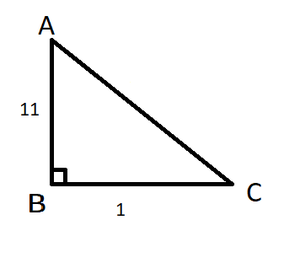Using Pythagoras Theorem, in △ABC,

(Hypotenuse)2 = (Perpendicular)2 + (Base)2

AC2 = AB2 + BC2

AC2 = (11)2 + (1)2

AC2 = 121  + 1

= 122

AC = √122units

Now,

sinθ = Perpendicular/Hypotenuse = AB/AC = 11/ √122

cosθ = Base/Hypotenuse = BC/AC = 1/√122

cotθ = 1/tanθ = 1/11

secθ = 1/cosθ = √122/1

cosecθ = 1/sinθ = √122/11

### (iv) sinθ = 11/15

Solution:

sinθ = 11/15 = Perpendicular/Hypotenuse

Draw a right-angled △ABC in which ∠B is = 90°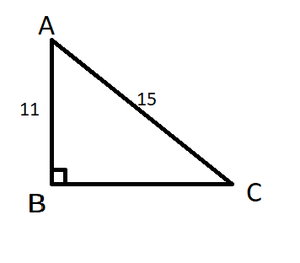Using Pythagoras Theorem, in △ABC,

(Hypotenuse)2 = (Perpendicular)2 + (Base)2

AC2 = AB2 + BC2

(15)2 = (11)2 + (BC)2

225 = 121 + (BC)2

(BC)2 = 104

BC = 2√26

Now,

cosθ = Base/Hypotenuse = BC/AC = 2√26/15

tanθ = AB/BC = 11/ 2√26

cotθ = 1/tanθ = 2√26/11

secθ = 1/cosθ = 15/ 2√26

cosecθ = 1/sinθ = 15/11

### (v) tan α = 5/12

Solution:

tan α = 5/12 = Perpendicular/Base

Draw a right-angled △ABC in which ∠B is = 90°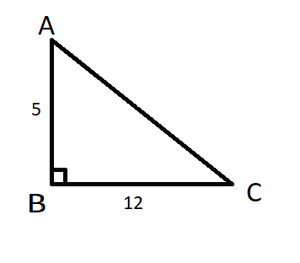Using Pythagoras Theorem, in △ABC,

(Hypotenuse)2 = (Perpendicular)2 + (Base)2

AC2 = AB2 + BC2

(AC)2 = (12)2 + (25)2

(AC)2 = 144 + 25

(AC)2 = 169

AC = √169 = 13 units

Now,

sin α = Perpendicular/Hypotenuse = AB/AC = 5/13

cos α = Base/Hypotenuse = BC/AC = 12/13

cot α = 1/tan α = 12/5

sec α = 1/cos α = 13/12

cosec α = 1/sin α = 13/5

### (vi) sinθ = √3/2

Solution:

sinθ = √3/2 = Perpendicular/Hypotenuse

Draw a right-angled △ABC in which ∠B is = 90°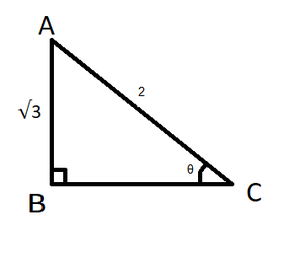Using Pythagoras Theorem, in △ ABC,

(Hypotenuse)2 = (Perpendicular)2 + (Base)2

AC2 = AB2 + BC2

(2)2 = (√3​)2 + (BC)2

4 = 3 + (BC)2

(BC)2 = 4 – 3 = 1

BC = 1 units

Now,

cosθ = Base/Hypotenuse = BC/AC = 1/2

tanθ = AB/BC = √3/1

cotθ = 1/tanθ = 1/√3

secθ = 1/cosθ = 2/1

cosecθ = 1/sinθ = 2/√3

### (vii) cosθ = 7/25

Solution:

cosθ = 7/25 = Base/Hypotenuse

Draw a right-angled △ABC in which ∠B is = 90°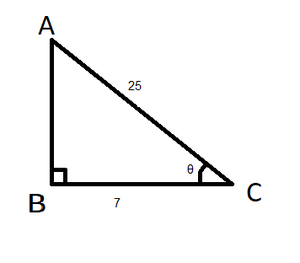Using Pythagoras Theorem, in △ ABC,

(Hypotenuse)2 = (Perpendicular)2 + (Base)2

AC2 = AB2 + BC2

(25)2 = (AB)2 + (7)2

625 = (AB)2 + 49

(AB)2 = 625 – 49 = 576

AB = √576 = 24 units

Now,

sinθ = Perpendicular/Hypotenuse = AB/AC = 24/25

tanθ = Perpendicular/Base = AB/BC = 24/7

cotθ = 1/tanθ = 7/24

secθ = 1/cosθ = 25/7

cosecθ = 1/sinθ = 25/24

### (viii) tanθ = 8/15

Solution:

tanθ = 8/15 = Perpendicular/Base

Draw a right-angled △ABC in which ∠B is = 90°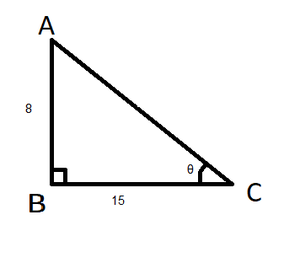Using Pythagoras Theorem, in △ ABC,

(Hypotenuse)2 = (Perpendicular)2 + (Base)2

AC2 = AB2 + BC2

(AC)2 = (8)2 + (15)2

(AC)2 = 64 + 225

AC = √289 = 17

Now,

sinθ = Perpendicular/Hypotenuse = AB/AC = 8/17

cosθ = Base/Hypotenuse = BC/AC = 15/17

cotθ = 1/tanθ = 15/8

secθ = 1/cosθ = 17/15

cosecθ = 1/sinθ = 17/8

### (ix) cotθ = 12/5

Solution:

cotθ = 12/5 = Base/Perpendicular

Draw a right-angled △ABC in which ∠B is = 90°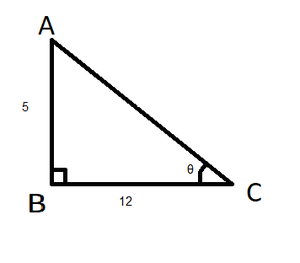Using Pythagoras Theorem, in △ABC,

(Hypotenuse)2 = (Perpendicular)2 + (Base)2

AC2 = AB2 + BC2

(AC)2 = (5)2 + (12)2

(AC)2 = 25 + 144

(AC)2 = 169

AC = √169 = 13 units

Now,

sinθ = Perpendicular/Hypotenuse = AB/AC = 5/13

cosθ = Base/Hypotenuse = BC/AC = 12/13

tanθ = 1/tanθ = 5/12

secθ = 1/cosθ = 13/12

cosecθ = 1/sinθ = 13/5

### (x) secθ = 13/5

Solution:

secθ = 13/5 = Hypotenuse/Base

Draw a right-angled △ABC in which ∠B is = 90°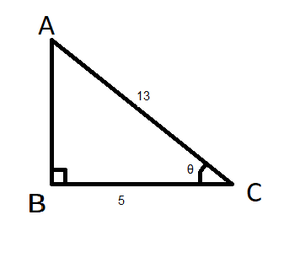Using Pythagoras Theorem, in △ABC,

(Hypotenuse)2 = (Perpendicular)2 + (Base)2

AC2 = AB2 + BC2

(13)2 = (AB)2 + (5)2

169 = (AB)2 + 25

(AB)2 = 169 – 25 = 144

AB = √144 = 12 units

Now,

sinθ = Perpendicular/Hypotenuse = AB/AC = 12/13

tanθ = Perpendicular/Base = AB/BC = 12/5

cotθ = 1/tanθ = 5/12

cosθ = 1/secθ = 5/13

cosecθ = 1/sinθ = 13/12

### (xi) cosecθ = √10

Solution:

cosecθ = √10/1 = Hypotenuse/Perpendicular

Draw a right-angled △ABC in which ∠B is = 90°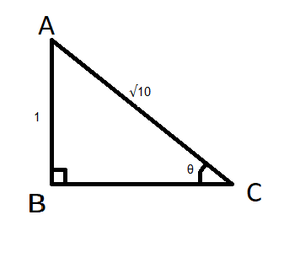Using Pythagoras Theorem, in △ ABC,

(Hypotenuse)2 = (Perpendicular)2 + (Base)2

AC2 = AB2 + BC2

(√10)2 = (1)2 + (BC)2

10 = 1 + (BC)2

(BC)2 = 10 – 1 = 9

BC = √9 = 3

Now,

sinθ = Perpendicular/Hypotenuse = AB/AC = 1/√10

cosθ = Base/Hypotenuse = BC/AC = 3/√10

tanθ = Perpendicular/Hypotenuse = AB/BC = 1/3

cotθ = 1/tanθ = 3/1 = 3

secθ = 1/cosθ = √10/3

### (xii) cosθ = 12/15

Solution:

cosθ = 12/15 = Base/Hypotenuse

Draw a right-angled △ABC in which ∠B is = 90°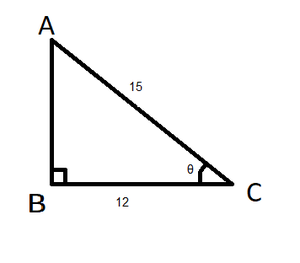Using Pythagoras Theorem, in △ABC,

(Hypotenuse)2 = (Perpendicular)2 + (Base)2

AC2 = AB2 + BC2

(15)2 = (AB)2 + (12)2

225 = (AB)2 + 144

(AB)2 = 225 – 144 = 81

AB = √81 = 9 units

Now,

sinθ = Perpendicular/Hypotenuse = AB/AC = 9/15

tanθ = Perpendicular/Base = AB/BC = 9/12

cotθ = 1/tanθ = 12/9

secθ = 1/cosθ = 15/12

cosecθ = 1/sinθ = 15/9

### (ii) sin C, cos C

Solution:

Given:

In right-angled ΔABC,

AB = 24 cm, BC = 7 cm. ∠B = 90°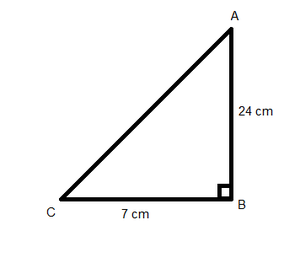Using Pythagoras Theorem

AC2 = AB2 + BC2

AC2 = 242 + 72 = 576 + 49

AC2 = 625

AC = √625 = 25cm

Now,

(i) sinA = BC/AC = 7/25

cosA = AB/AC = 24/25

(ii) sinC = AB/AC = 24/25

cosC = BC/AC = 7/25

### Question 3. In the figure, find tan P and cot R. Is tan P = cot R?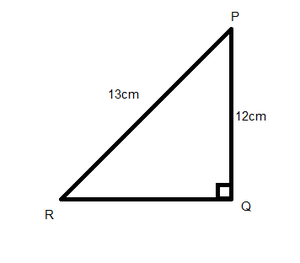Solution:

Using Pythagoras Theorem

PR2 = PQ2 + QR2

132 = 122 + QR2

QR2 = 169 – 144 = 25

QR = √25 = 5 cm

Now,

tan P = Perpendicular/Base = QR/PQ = 5/2

cot R = Base/Perpendicular = QR/PQ = 5/2

Yes, tanP = cot R

### Question 4. If sin A = 9/41, compute cos A and tan A.

Solution:

Given, sinA = 9/41 = Perpendicular/Hypotenuse

Draw a △ ABC where ∠B = 90°, BC = 9, AC = 41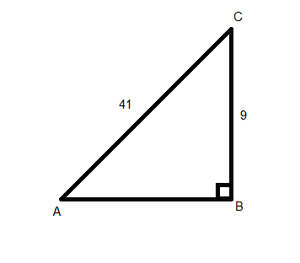Using Pythagoras Theorem

AC2 = AB2 + BC2

BC2 = 412 – 92 = 1681 – 81

BC2 = 1600

BC = √1600 = 40

Now, cos A = Base/Hypotenuse = AB/AC = 40/41

tan A = Perpendicular/Base = BC/AB = 9/40

### Question 5. Given 15 cot A = 8, find sin A and sec A.

Solution:

Given, 15 cot A = 8

cot A = 8/15 = Base/Perpendicular

Draw a △ ABC where ∠B = 90°, AB = 8, BC = 15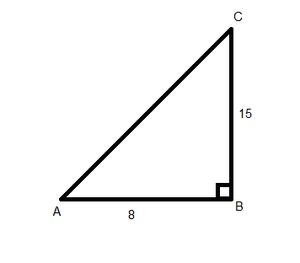Using Pythagoras Theorem

AC2 = AB2 + BC2

AC2 = 82 + 152 = 64 + 225

AC2 = 289

AC = √289 = 17

Now,

sin A = Perpendicular/Hypotenuse = BC/AC = 15/17

sec A = Hypotenuse/Base = AC/AB = 17/8

### Question 6. In ΔPQR, right-angled at Q, PQ = 4 cm and RQ = 3 cm. Find the values of sin P, sin R, sec P, and sec R.

Solution:

In right-angled ΔPQR,

∠Q = 90°, PQ = 4cm, RQ = 3cm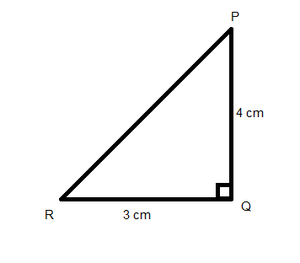Using Pythagoras Theorem

PR2 = PQ2 + QR2

PR2 = 42 + 32 = 16 + 9

PR2 = 25

PR = √25 =5

Now,

sin P = Perpendicular/Hypotenuse = RQ/PR = 3/5

sin R = Perpendicular/Hypotenuse = PQ/PR = 4/5

sec P = Hypotenuse/Base = PR/PQ = 5/4

sec R = Hypotenuse/Base = PR/RQ = 5/3

My Personal Notes arrow_drop_up